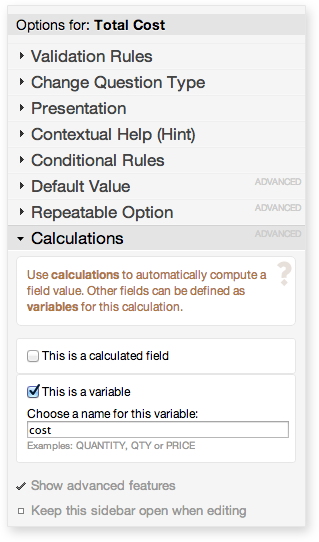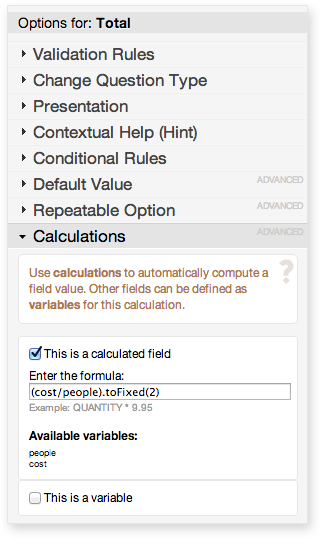Select Page

If you use calculations on your form, you may find that you want to limit the calculation’s answer to a certain number of decimal places. This is especially useful when presenting prices or averages.

Limiting the number of decimal places is actually quite easy and requires only a little javascript.

Define variables

First, make sure you’ve defined the variables you want to use in the calculation. Give them meaningful names so you can easily remember what they represent. For this example, let’s consider that we’re planning a trip. We know how many people are coming and the total cost of the trip, so we want to find the cost per person and round it to the nearest cent.

Our first variable is the number of people.Next, define the total cost variable.Create your calculation field, then determine the formula you need to use. In this case, that will be cost/people. To round to two decimal places, add .toFixed(2) to the end of the formula, so the final formula looks like this:

(cost/people).toFixed(2)Other Calculations

FormAssembly enables you to use many different calculations on your forms. For more information, see our Support Documentation and our previous blog post on using calculations and repeatable sections.